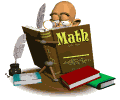### MATH

• This year we will be using the New York State Math Modules along with supplemental materials. These materials integrate the Common Core Learning Standards. We will be covering (1) ratios and unit rates; (2) arithmetic operations including dividing by a fraction; (3) rational numbers; (4) expressions and equations; (5) area, surface area, and volume problems; and (6) statistics. There will be quizzes, mid-topic and end-of-topic assessments along with nightly homework assignments.

Excel Sample Data

Sample Data Set A